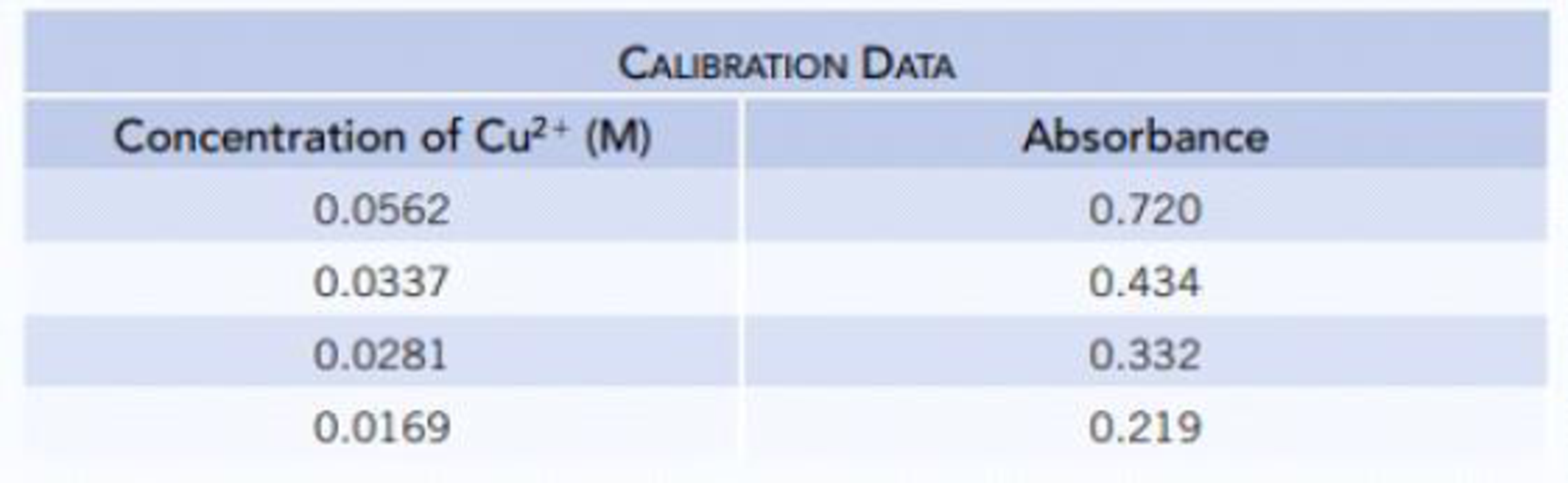Chapter 4.9, Problem 4.14CYU

Chapter
Section
Textbook Problem

A solution of copper(II) ions has an absorbance of 0.418 when measured at 645 nm in a 1.0-cm cell. Using the following data, calculate the concentration of copper(II) ions in the unknown solution.Interpretation Introduction

Interpretation: The concentration of copper (II) ions in the given unknown solution should be calculated.

Concept Introduction:

• Concentration of solutions can be expressed in various terms; molarity is one such concentration expressing term.
Explanation

First the given absorbance and the concentration data are plotted as follows,

When x=0y is 0.011, therefore the y intercept is 0.011.

From this the equation of straight line the concentration is calculated as follows,

y =  mx + cy =13x + 0

Still sussing out bartleby?

Check out a sample textbook solution.

See a sample solution

The Solution to Your Study Problems

Bartleby provides explanations to thousands of textbook problems written by our experts, many with advanced degrees!

Get Started

Find more solutions based on key concepts